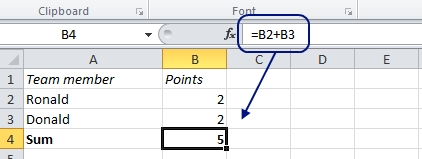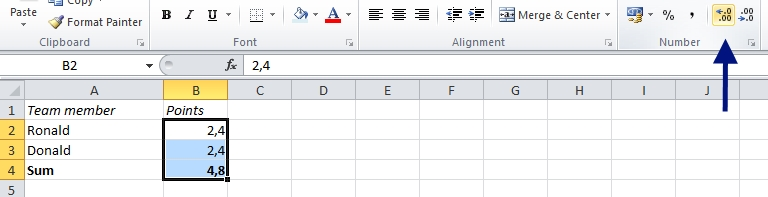# Excel: 2 + 2 = 5

Can 2 +2 be 5?

Yes, at least if you look at this example in Excel:Of course, Excel doesn’t make mistakes like that, so there must be an explanation. Let’s try to increase the number of decimals:As it turns out, the real calculation wasn’t 2 + 2; it just looked like that. It was actually 2,4 + 2,4 = 4,8, but when you decrease the number of shown decimals to none, Excel displays the nearest integer in the cell, and it looks like 2 + 2 = 5. The actual number behind doesn’t change.

More Excel oddities:

Are you using a non-English version of Excel? Click here for translations of the 100 most common functions.